132, My Street,
Kingston, New York 12401
United States
##### Phone Information
Phone: (+1) 234 567 89
Tel: +1-541-234-3010
We want to provide you with a great experience which is why we want to hear from you. Your feedback helps us bring you more of the events you love and the service you expect.

# How to Find VPP on Oscilloscope?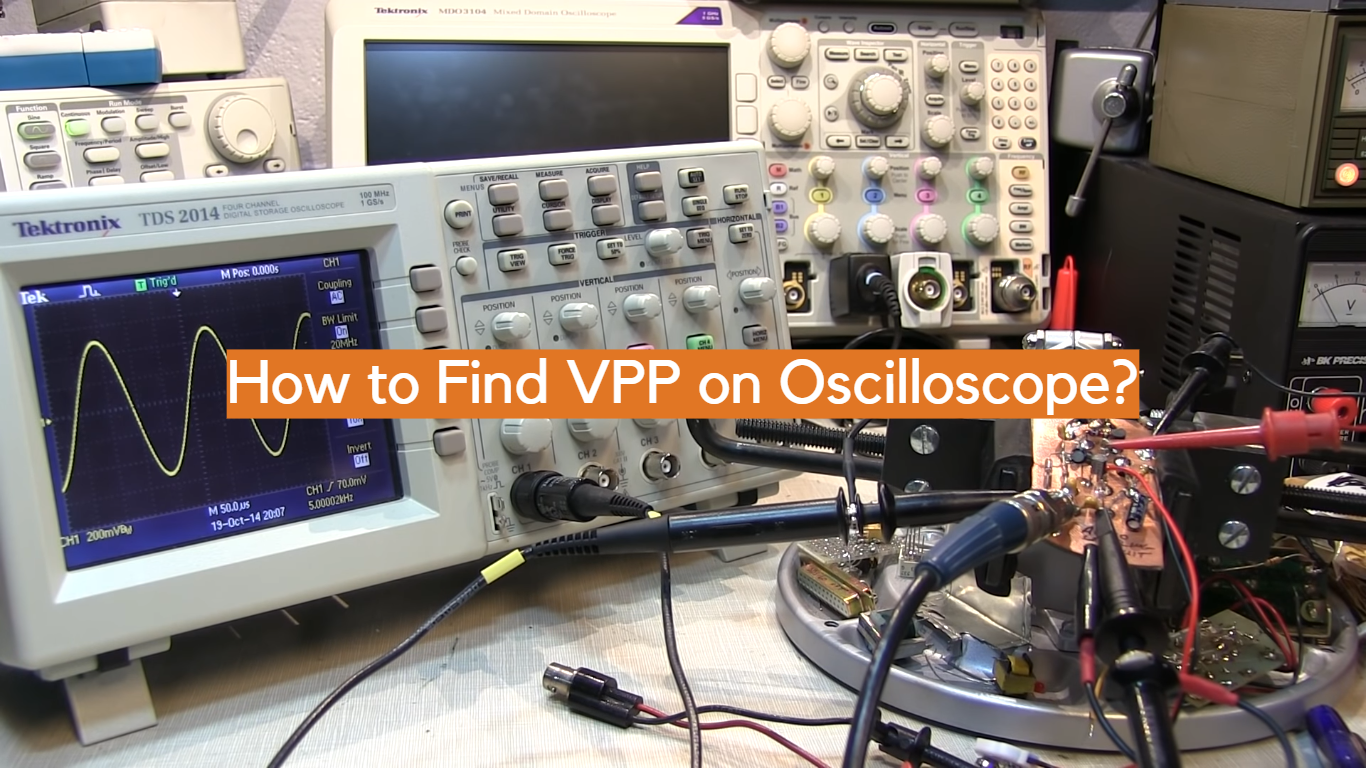An oscilloscope is an indispensable tool for engineers, technicians, and hobbyists working with electrical signals. It allows us to visualize and analyze waveforms, providing critical insights into various electrical phenomena.

One of the fundamental measurements an oscilloscope provides is the Voltage Peak-to-Peak (VPP), which characterizes the full amplitude of an AC waveform, including both its positive and negative peaks within a single cycle. Understanding how to find VPP on an oscilloscope is essential for accurate signal analysis, circuit troubleshooting, and ensuring optimal performance of electronic systems.

In this comprehensive guide, we will walk you through a step-by-step process to calculate VPP on an oscilloscope effectively. Whether you are a seasoned professional or a curious beginner, mastering this skill will empower you to interpret waveforms with confidence, identify irregularities, and make informed decisions in electronics design and testing.

Let’s delve into the world of oscilloscopes and unveil the secrets of calculating Voltage Peak-to-Peak amplitude for a deeper understanding of electrical signals.

## What is Peak-to-Peak Voltage (VPP)?

In the realm of electrical engineering and signal analysis, understanding voltage is fundamental. One crucial aspect of voltage measurement is the concept of “Peak-to-Peak Voltage” (VPP). VPP represents a significant parameter in characterizing alternating current (AC) and periodic waveforms. It refers to the difference between the highest positive peak and the lowest negative peak of a voltage waveform within a single cycle .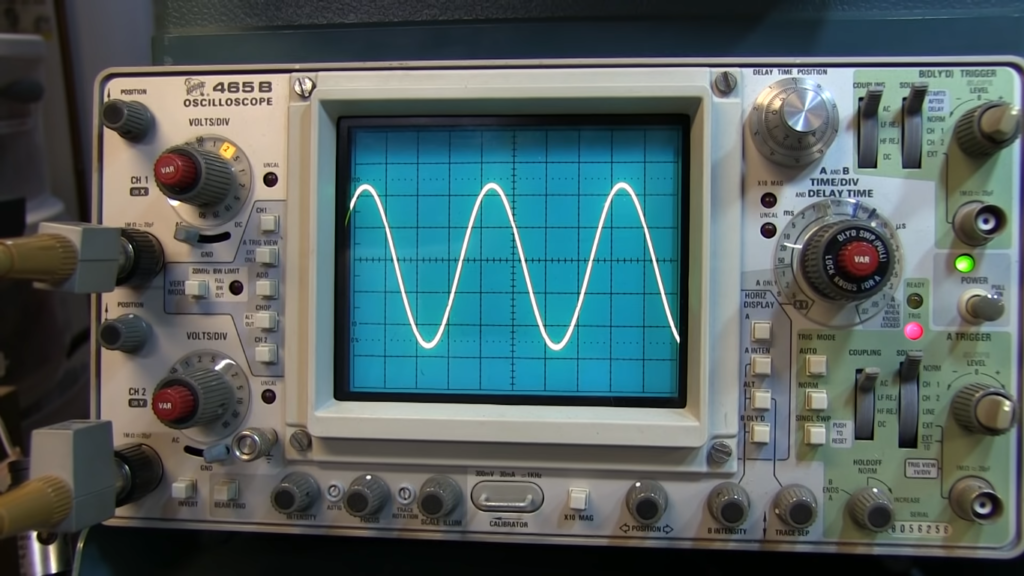In simple terms, the peak-to-peak voltage measures the full amplitude of an AC signal, encompassing both its positive and negative excursions. Engineers and technicians commonly use VPP when dealing with periodic waveforms like sine waves, square waves, or triangle waves. By determining the VPP value, they can better assess the range within which the voltage oscillates, providing valuable insights into the behavior and performance of electrical systems.

To calculate VPP, one must first identify the highest peak and lowest peak of the waveform during a complete cycle. Next, the absolute values of these two peaks are determined, and their difference results in the peak-to-peak voltage. Mathematically, it can be expressed as:

VPP = |Vmax| – |Vmin|

Where:

• VPP = Peak-to-Peak Voltage
• Vmax = Maximum positive peak voltage
• Vmin = Maximum negative peak voltage

The understanding of peak-to-peak voltage finds applications in various fields. In electronics, VPP helps in evaluating the capabilities of amplifiers and the voltage headroom they can handle without distortion. Additionally, when designing and testing electronic circuits, it assists in determining appropriate voltage levels for components to avoid voltage spikes or dips that could potentially damage the system.

Furthermore, in the field of telecommunications and audio engineering, peak-to-peak voltage plays a pivotal role. Understanding VPP helps ensure the accurate transmission and reproduction of audio signals, resulting in improved sound quality and fidelity. Moreover, in data communication, knowing the VPP of the transmitted signals is essential to prevent signal degradation and ensure reliable data transfer.

In industrial applications, peak-to-peak voltage is crucial for assessing the performance and health of motors and generators. It aids in diagnosing irregularities, identifying potential faults, and optimizing power consumption.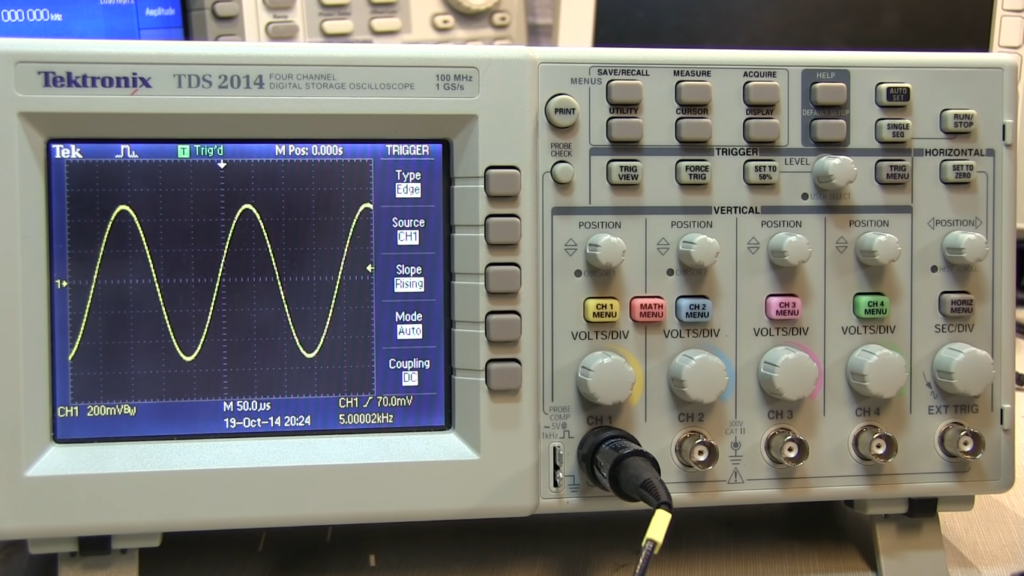## Examples of Peak-to-Peak Voltage

Peak-to-Peak Voltage (VPP) is a critical parameter used to measure the amplitude of alternating current (AC) waveforms. Here are some examples of peak-to-peak voltage in different electrical scenarios:

### 1) Sine Wave

A pure sine wave is a fundamental waveform commonly found in AC power systems and electronic circuits. The peak-to-peak voltage of a standard 120VAC household power supply can be calculated as follows:

VPP = |Vmax| – |Vmin| = |170.7V| – |(-170.7V)| = 341.4V

In this case, the VPP value of the sine wave is 341.4 volts.

### 2) Audio Signal

In audio engineering, understanding peak-to-peak voltage is crucial for maintaining the integrity of sound signals. Consider an audio signal with a peak voltage of 2V and a trough voltage of -2V. The peak-to-peak voltage would be:

VPP = |Vmax| – |Vmin| = |2V| – |(-2V)| = 4V

The peak-to-peak voltage of this audio signal is 4 volts.

### 3) Square Wave

A square wave is a type of non-sinusoidal waveform characterized by sharp transitions between high and low voltage levels.

Suppose a square wave has a high level of 5V and a low level of 0V. The peak-to-peak voltage can be calculated as:

VPP = |Vmax| – |Vmin| = |5V| – |0V| = 5V

The peak-to-peak voltage of this square wave is 5 volts.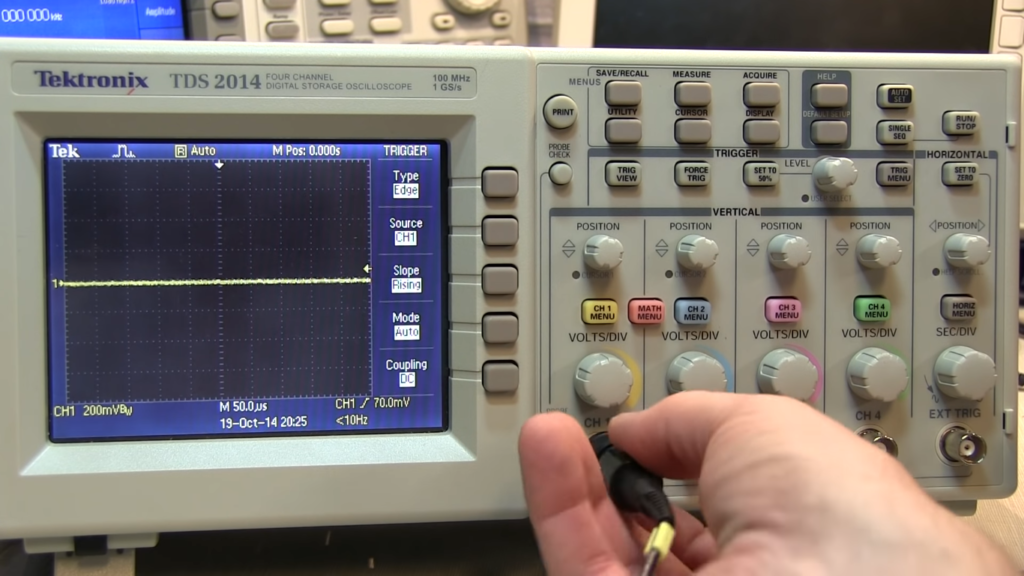### 4) Triangle Wave

A triangle wave is another periodic waveform with linearly increasing and decreasing voltage levels. If a triangle wave has a maximum positive peak of 3V and a maximum negative peak of -3V, the peak-to-peak voltage is:

VPP = |Vmax| – |Vmin| = |3V| – |(-3V)| = 6V

The peak-to-peak voltage of this triangle wave is 6 volts.

### 5) Electrical Motors

In industrial applications, electrical motors are essential components. Monitoring the peak-to-peak voltage across the motor windings can help identify potential issues. For instance, if a motor has a peak voltage of 400V and a trough voltage of -400V, the peak-to-peak voltage is:

VPP = |Vmax| – |Vmin| = |400V| – |(-400V)| = 800V

The peak-to-peak voltage across the motor is 800 volts .

## What Does VPP Mean on an Oscilloscope?

On an oscilloscope, VPP stands for “Voltage Peak-to-Peak”. A measurement parameter represents the total amplitude of an electrical signal displayed on the oscilloscope screen. The VPP measurement on an oscilloscope indicates the voltage difference between the highest positive peak and the lowest negative peak of the waveform within one complete cycle.

Oscilloscopes are essential tools in electrical engineering, electronics, and various scientific fields for visualizing and analyzing electrical signals. They display waveforms graphically, with voltage represented on the vertical axis and time on the horizontal axis.

To measure VPP on an oscilloscope, follow these steps:

1. Signal Connection: Connect the electrical signal you want to measure to the input channel of the oscilloscope. Depending on the type of signal, you may need appropriate probes or adapters;
2. Vertical Scaling: Adjust the vertical scaling (volts per division) of the oscilloscope so that the waveform of interest fits comfortably within the visible area on the screen;
3. Triggering: Set the triggering mode and level to stabilize the waveform on the display. This ensures that the waveform remains stationary for accurate measurements;
4. Measurement: Locate the “Measure” or “Utility” menu on your oscilloscope and select “VPP” as the measurement type. The oscilloscope will then automatically calculate and display the peak-to-peak voltage value of the waveform;
5. Read the Value: The VPP measurement will be shown on the screen, typically in volts. This value represents the full amplitude of the waveform, including both its positive and negative excursions ;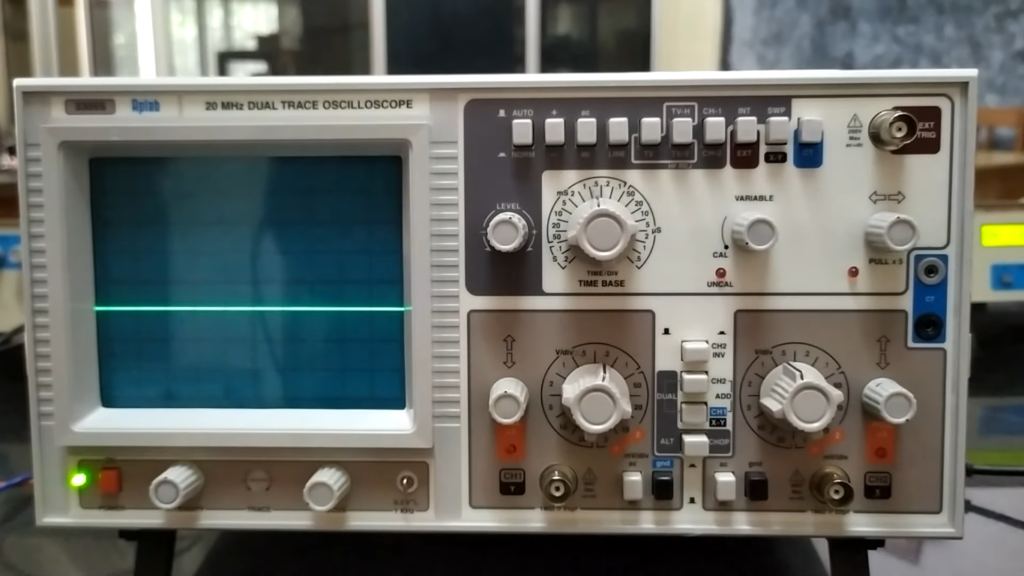VPP is a valuable measurement on an oscilloscope, as it provides essential information about the voltage range and amplitude of the signal under observation. Engineers and technicians use this parameter to assess the signal’s behavior, identify irregularities, ensure it is within the desired voltage limits, and troubleshoot issues in electronic circuits or electrical systems.

In addition to VPP, oscilloscopes offer various other measurement parameters, such as peak voltage (Vmax), minimum voltage (Vmin), root-mean-square (RMS) voltage, frequency, and more. These measurements collectively help in comprehensive signal analysis and accurate characterization of electrical waveforms.

## How to Find VPP on Oscilloscope:

### Step 1: Do The Adjustments

Before making any measurements on an oscilloscope, it is crucial to set up the instrument correctly for accurate results. Follow these initial adjustments:

1. Connect the Signal: Begin by connecting the electrical signal you want to measure to the input channel of the oscilloscope. Depending on the signal type, you may need appropriate probes or adapters;
2. Vertical Scaling: Adjust the vertical scaling (volts per division) on the oscilloscope so that the waveform appears clearly and does not extend beyond the visible area of the screen. This ensures that the entire waveform is visible and easy to analyze;
3. Timebase Setting: Set the timebase (time per division) on the oscilloscope according to the frequency of the signal. Adjust it so that you can see at least a few cycles of the waveform on the screen simultaneously;
4. Triggering: Proper triggering stabilizes the waveform on the display. Set the triggering mode (edge, pulse, etc.) and adjust the trigger level to ensure a stable display of the signal;

### Step 2: Measure The Vertical Distance

The vertical distance of a waveform represents its voltage level. To measure VPP, we need to find the vertical distance between the highest positive peak and the lowest negative peak of the waveform.

1. Adjust Vertical Position: If needed, adjust the vertical position of the waveform so that it is centered vertically on the screen. This ensures that the peaks are equally visible and easily measurable;
2. Identify Peaks: Use the vertical grid lines on the oscilloscope display to identify the highest positive peak and the lowest negative peak of the waveform. These points are where the signal reaches its maximum positive and negative voltages, respectively;
3. Read Vertical Values: Look at the vertical scale on the side of the screen to determine the voltage value corresponding to each peak. Note down the voltage values of both the positive and negative peaks;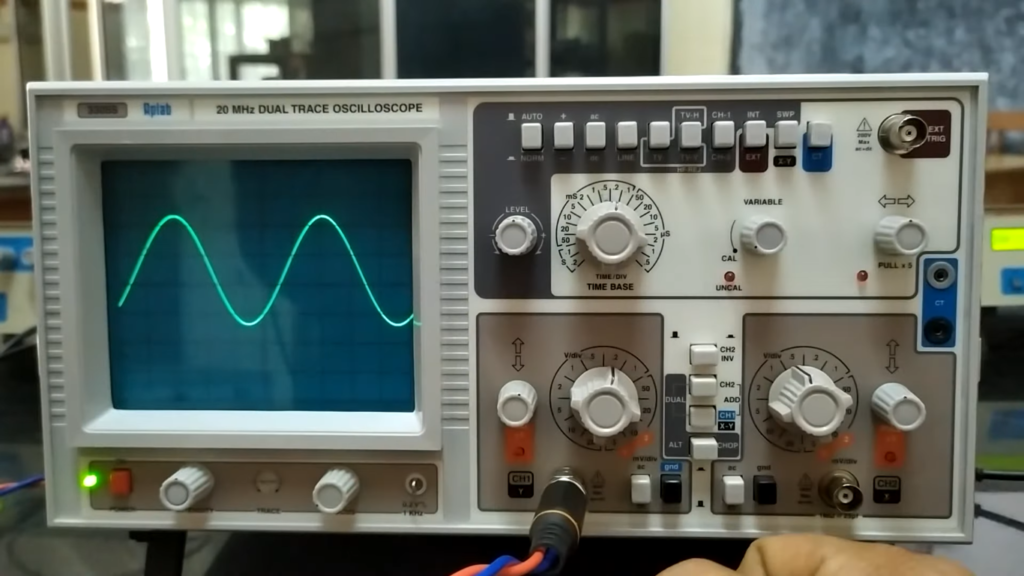### Step 3: Calculate The Peak Voltage

With the vertical distances of the positive and negative peaks identified, we can now calculate the Voltage Peak-to-Peak (VPP) using the following formula:

VPP = |Vmax| – |Vmin|

Where:

• VPP is the Voltage Peak-to-Peak
• Vmax is the maximum positive peak voltage
• Vmin is the maximum negative peak voltage

Take Absolute Values: Since VPP requires the difference between the absolute values of the peaks, it is essential to use the absolute values of Vmax and Vmin in the calculation;
Calculate VPP: Subtract the absolute value of Vmin from the absolute value of Vmax to obtain the Voltage Peak-to-Peak (VPP) value;

### Step 4: Record and Analyze the Result

Record the VPP value you obtained from the calculation. This value represents the full amplitude of the waveform, including both its positive and negative excursions. Understanding the VPP is valuable for various applications, such as assessing the behavior of electrical signals, identifying irregularities or distortions, and ensuring the signals are within desired voltage limits.

By knowing the VPP of a waveform, engineers and technicians can make informed decisions when designing and testing electronic circuits, troubleshooting electrical systems, or optimizing the performance of electrical equipment.

## How Do You Calculate Peak to Peak Amplitude From Oscilloscope:

### Peak-to-Peak Amplitude Measurement

To calculate the peak-to-peak amplitude from an oscilloscope, you need to follow these steps:

### Step 1: Signal Connection

Connect the electrical signal of interest to the input channel of the oscilloscope. Use appropriate probes or adapters depending on the type of signal being measured.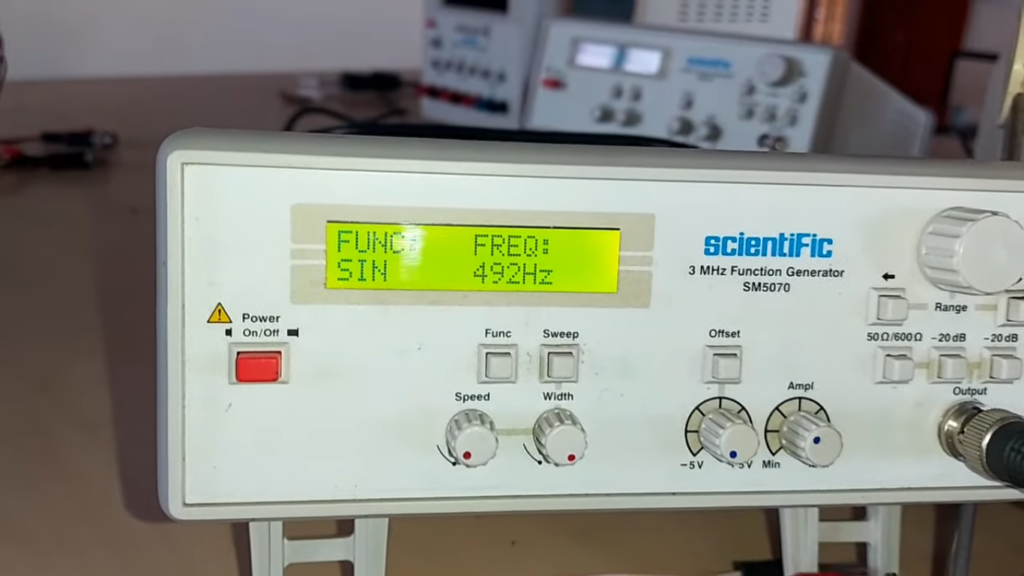### Step 2: Vertical Scaling

Adjust the vertical scaling (volts per division) on the oscilloscope so that the entire waveform is visible on the screen. This ensures that the peaks can be accurately measured.

### Step 3: Timebase Setting

Set the timebase (time per division) on the oscilloscope to visualize several cycles of the waveform simultaneously. This aids in accurately identifying the peaks.

### Step 4: Triggering

Ensure proper triggering to stabilize the waveform on the display. Adjust the trigger mode and level to achieve a steady display of the signal.

### Step 5: Identify Peaks

Use the vertical grid lines on the oscilloscope display to identify the highest positive peak and the lowest negative peak of the waveform. These points represent the maximum positive and negative voltages, respectively.

### Step 6: Measure Vertical Distance

Find the vertical distance between the positive and negative peaks on the oscilloscope screen. This vertical distance represents the peak-to-peak amplitude .

### Step 7: Calculate Peak-to-Peak Amplitude

The peak-to-peak amplitude (A_PP) is calculated by taking the difference between the maximum positive and negative voltage values:

A_PP = |Vmax| – |Vmin|

Where:

• A_PP is the Peak-to-Peak Amplitude
• Vmax is the maximum positive peak voltage
• Vmin is the maximum negative peak voltage

## Is VPP the Same as Amplitude?

The peak-to-peak amplitude, or VPP, is defined as the difference between the largest and smallest voltages in a waveform.

Amplitude, on the other hand, is a measure of the magnitude of a signal, typically measured as the maximum displacement from zero volts.

In some cases, VPP and amplitude may be equivalent, but this depends on the specific context and definition being used. It is therefore important to clarify the definition being used in any given situation to avoid confusion.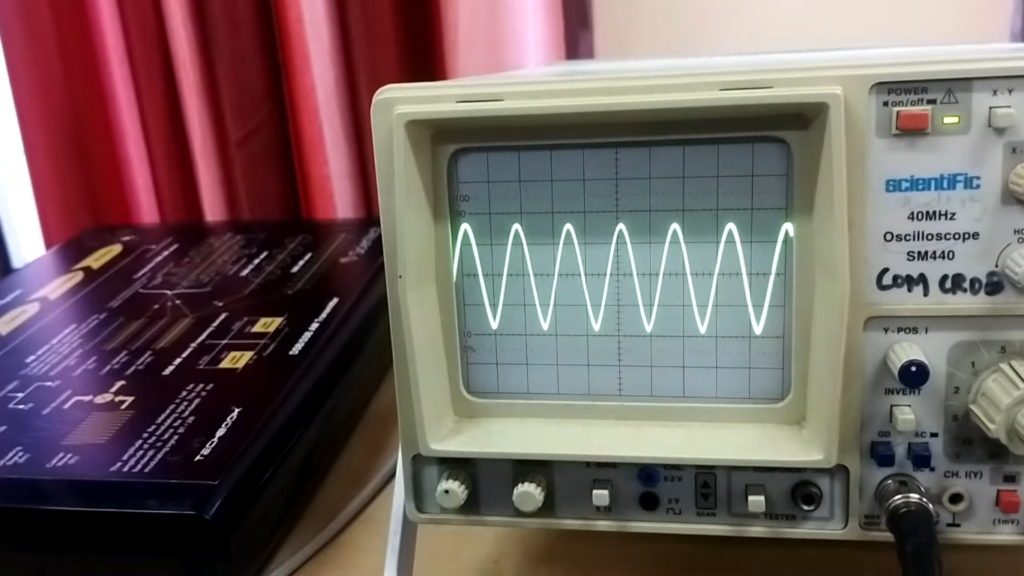While peak-to-peak voltage (VPP) and amplitude are related, they are not the same. VPP represents the total voltage swing from the highest positive peak to the lowest negative peak of a waveform. On the other hand, amplitude refers to the magnitude of the voltage from the baseline to the peak of a waveform (whether positive or negative) without considering the full voltage swing.

In mathematical terms:

VPP = |Vmax| – |Vmin|
Amplitude = |Vmax| or |Vmin|

The amplitude can be half of the peak-to-peak voltage when considering either the positive or negative half of the waveform. Understanding the distinction between VPP and amplitude is essential when interpreting oscilloscope measurements and performing signal analysis.

## FAQ:

### 1. How to Calculate Peak-to-Peak Voltage from Peak Voltage?

Peak-to-Peak Voltage (VPP) represents the total voltage swing of a waveform from its highest positive peak to its lowest negative peak. To calculate VPP from Peak Voltage (Vmax), follow these steps:

VPP = 2 * Vmax

Since peak voltage refers to the maximum positive peak of the waveform, doubling this value gives us the full voltage swing, considering both the positive and negative excursions.

### 2. How to Calculate Peak-to-Peak Voltage from RMS Voltage?

RMS (Root Mean Square) Voltage represents the equivalent DC voltage that would produce the same amount of power dissipation in a resistive load as the original AC waveform. To calculate VPP from RMS Voltage (VRMS), follow these steps:

VPP = 2 * √2 * VRMS

Multiplying the RMS voltage by 2√2 gives us the approximate peak-to-peak voltage for a sinusoidal waveform.

### 3. How to Calculate Peak-to-Peak Voltage from Average Voltage?

Average Voltage represents the mean voltage value of an AC waveform over one complete cycle. To calculate VPP from Average Voltage (Vavg), follow these steps:

VPP = 2 * (Vavg – Vmin)

Subtract the minimum voltage (Vmin) from the average voltage and then double the result to obtain the peak-to-peak voltage.

### 4. What is VPP in Oscilloscope?

In an oscilloscope, VPP stands for “Voltage Peak-to-Peak”. The measurement parameter represents the total amplitude of an electrical signal displayed on the oscilloscope screen. VPP indicates the voltage difference between the highest positive peak and the lowest negative peak of the waveform within one complete cycle.

### 5. What is the Peak-to-Peak Amplitude of Oscilloscope?

The Peak-to-Peak Amplitude on an oscilloscope is the same as VPP, representing the total voltage swing of a waveform from its highest positive peak to its lowest negative peak.

### 6. Is VPP the Same as Amplitude?

No, VPP (Voltage Peak-to-Peak) and amplitude are not the same. VPP represents the full voltage swing from the highest positive peak to the lowest negative peak of a waveform.

On the other hand, amplitude refers to the magnitude of the voltage from the baseline to the peak of a waveform (whether positive or negative) without considering the full voltage swing. Mathematically, VPP = 2 * Amplitude.

### 7. How do you Calculate RMS?

To calculate RMS (Root Mean Square) of a set of values, follow these steps:

1. Square each value in the set;
2. Calculate the average of the squared values;
3. Take the square root of the average;

### 8. How do you calculate peak voltage in 3 phase?

In a 3-phase system, the peak voltage is calculated differently depending on whether it is a line-to-line or line-to-neutral voltage.

For line-to-line peak voltage (Vpp_LL), use the formula:

Vpp_LL = √3 * Vrms

For line-to-neutral peak voltage (Vpp_LN), use the formula:

Vpp_LN = 2 * Vrms

### 9. How do you calculate VDC from a full-wave rectifier?

In a full-wave rectifier, the output voltage (VDC) can be calculated from the peak voltage (Vmax) of the rectified waveform using the formula:

VDC = Vmax – Vd

Where Vd is the voltage drop across the diode(s) in the rectifier circuit.

### 10. What is VDC in Rectifier?

VDC stands for “DC Voltage” in a rectifier. It represents the average output voltage of a rectifier circuit after converting an AC input to DC output.

### 11. What is VDC and VAC?

VDC stands for “DC Voltage”, representing a constant voltage level without any alternating component. VAC stands for “AC Voltage”, representing a voltage that changes polarity periodically, oscillating between positive and negative values. Both VDC and VAC are essential concepts in electrical engineering, used to describe different types of voltage sources and signals.

### 12. What is VPP, and why is it important to measure it on an oscilloscope?

VPP stands for “peak-to-peak voltage” and represents the voltage difference between the highest peak and lowest valley of an AC signal over one complete cycle. It is essential to measure VPP on an oscilloscope to understand the amplitude of a signal accurately, which is crucial for analyzing and troubleshooting electronic circuits.

### 13. How do I set up my oscilloscope to measure VPP?

To measure VPP on an oscilloscope, follow these steps:

1. Ensure your oscilloscope is properly calibrated and functioning;
2. Set the vertical scale and position to accommodate the signal without clipping or distortion;
3. Choose the appropriate coupling mode (AC coupling for AC signals);

If dealing with repetitive signals, use the “Auto” trigger mode; for single-shot events, use “Single” or “Normal” trigger modes.

### 14. Can I measure VPP on both AC and DC signals?

VPP measurement is relevant only for AC signals, as DC signals do not oscillate and have a VPP of zero. For DC signals, you can measure other parameters, such as DC voltage (VDC).

### 15. How do I manually calculate VPP on the oscilloscope if it doesn’t have an automated measurement function?

If your oscilloscope doesn’t have an automated VPP measurement function, you can manually calculate it. Identify the highest peak and lowest valley on the waveform and count the number of divisions between them on the vertical scale. Multiply this number by the voltage per division (V/div) setting to get the VPP.

### 16. My signal appears noisy on the oscilloscope. How can I get an accurate VPP measurement?

Noisy signals can affect measurement accuracy. Try increasing the oscilloscope’s bandwidth or averaging multiple waveforms to reduce noise. Check the grounding and connections to minimize noise interference.

### 17. Can I measure VPP on high-frequency signals?

Yes, you can measure VPP on high-frequency signals, but you need an oscilloscope with sufficient bandwidth to accurately capture the waveform. Lower bandwidth scopes may distort high-frequency signals, leading to inaccurate measurements.

### 18. How often should I calibrate my oscilloscope to maintain measurement accuracy?

It is recommended to calibrate your oscilloscope regularly, especially if you rely on precise measurements. The calibration interval can vary based on usage and the manufacturer’s recommendations. Some may need calibration annually, while others might require it more frequently.

### 19. What maintenance is required for my oscilloscope and its accessories?

Regular maintenance is essential for the proper functioning of your oscilloscope. Clean the screen, probes, and connectors regularly. Inspect them for any signs of wear or damage, and replace or repair as needed. Store your oscilloscope and accessories in a clean and dry environment when not in use.

### 20. Can I use the oscilloscope’s AC coupling mode for all AC signals?

While AC coupling is suitable for most AC signals, it may not be appropriate if the signal has a significant DC offset. In such cases, you may need to use the DC coupling mode to visualize the entire waveform accurately.

### 21. Are there any precautions I should take while using an oscilloscope to measure VPP?

Yes, some precautions to keep in mind are:

• Avoid exceeding the voltage rating of the oscilloscope and probes to prevent damage;
• Use proper grounding and connections to avoid noise and interference;
• Double-check that the oscilloscope settings (such as voltage scales and time scales) are appropriate for the signal you are measuring;

### 22. Can I measure VPP on a distorted or unstable signal?

It can be challenging to measure VPP accurately on distorted or unstable signals. In such cases, try using the oscilloscope’s “Normal” trigger mode to capture a stable waveform or average multiple waveforms to reduce noise.

### 23. What are the units for VPP measurement?

The units for VPP measurement are volts (V) or millivolts (mV), depending on the vertical scale setting of your oscilloscope.

### 24. Can I measure VPP on a digital oscilloscope?

Yes, digital oscilloscopes are equipped with various measurement functions, including VPP. You can measure VPP on both analog and digital oscilloscopes, either manually or using automated measurement options.

### 25. What should I do if I’m unsure about the oscilloscope’s settings or how to interpret the results?

If you are unsure about the oscilloscope’s settings or how to interpret the measurements, refer to the oscilloscope’s user manual for guidance. Additionally, online resources and tutorials can provide valuable insights and practical tips for oscilloscope usage. If needed, seek help from experienced colleagues or professionals in the field.